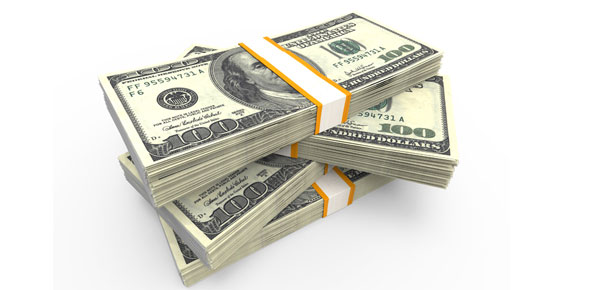# Nominal And Real Values

Approved & Edited by ProProfs Editorial Team
At ProProfs Quizzes, our dedicated in-house team of experts takes pride in their work. With a sharp eye for detail, they meticulously review each quiz. This ensures that every quiz, taken by over 100 million users, meets our standards of accuracy, clarity, and engagement.
| Written by KarolinaKaz
K
KarolinaKaz
Community Contributor
Quizzes Created: 1 | Total Attempts: 108
Questions: 10 | Attempts: 108Settings• 1.

### The ______value of a good is its value in terms of money

Explanation
The term "nominal" refers to the value of a good in terms of money. It signifies the face value or the stated value of an item, without taking into account factors such as inflation or market conditions. In other words, the nominal value represents the price or worth of a good as it is stated or recorded, rather than its actual or adjusted value.

Rate this question:

• 2.

### The ________ value of a good is its value in terms of other goods

Explanation
The real value of a good refers to its value in terms of actual, tangible resources such as money or physical assets. On the other hand, the relative value of a good refers to its value in comparison to other goods or services. In this context, the question is asking for the value of a good in terms of other goods, indicating that the answer is both real and relative.

Rate this question:

• 3.

### Inflation can affect the real/nominal price

• A.

True

• B.

False

A. True
Explanation
Inflation refers to the general increase in prices of goods and services over time. It affects both the real and nominal prices. The nominal price is the price at which a good or service is sold, while the real price takes into account the effect of inflation on purchasing power. As inflation increases, the nominal price of goods and services also tends to increase. This means that the real price, adjusted for inflation, may remain the same or even decrease. Therefore, it is true that inflation can affect the real/nominal price.

Rate this question:

• 4.

### The "real" price may also be called the ________ price

• A.

Street

• B.

Relative

• C.

Cash

• D.

Raw

B. Relative
Explanation
The "real" price refers to the price of a product or service in relation to other factors or variables. It is often used to compare prices in different contexts or adjust for inflation. Therefore, the term "relative" is an appropriate alternative to describe the "real" price, as it signifies the comparison or relationship between prices.

Rate this question:

• 5.

### Due to inflation, the dollar is worth more nowadays than it was before

• A.

True

• B.

False

B. False
Explanation
Inflation refers to the general increase in prices of goods and services over time, resulting in the decrease in the purchasing power of money. Therefore, the statement "Due to inflation, the dollar is worth more nowadays than it was before" is incorrect. Inflation causes the value of a currency to decrease, not increase. Hence, the correct answer is False.

Rate this question:

• 6.

### Real Wage = Nominal Wage / _____% Increase in Prices Since Base Year

• A.

1%

• B.

2%

• C.

5%

• D.

10%

A. 1%
Explanation
The real wage is calculated by dividing the nominal wage by the percentage increase in prices since the base year. In this case, the correct answer is 1% because it represents the percentage increase in prices. This means that the real wage is adjusted for a 1% increase in prices since the base year.

Rate this question:

• 7.

### Real prices can _________ even if nominal prices increase

decrease
dicrease
go down
lower
fall
Explanation
Real prices can decrease even if nominal prices increase. This is because real prices take into account the effect of inflation on the purchasing power of money. If nominal prices increase but the rate of inflation is higher, the real prices will actually decrease. Similarly, if nominal prices remain the same but the rate of inflation decreases, the real prices will also decrease. In both cases, the real prices go down, lower, or fall.

Rate this question:

• 8.

### The nominal price of 5 bananas is

• A.

\$600

• B.

5 oranges

• C.

Around \$3

• D.

A banana peel

C. Around \$3
Explanation
The given information states that the nominal price of 5 bananas is around \$3. This implies that each banana costs approximately \$0.60 (\$3 divided by 5).

Rate this question:

• 9.

### The real price of a water bottle is

• A.

\$0.25

• B.

A cactus

• C.

\$2

• D.

A granola bar

D. A granola bar
Explanation
The given options present the prices of a water bottle, a cactus, and a granola bar. The correct answer is "a granola bar" because it is the only option that provides a specific price, which is not given for the other items. Therefore, the real price of a water bottle remains unknown based on the given information.

Rate this question:

• 10.

### The "real" value of a good is basically

• A.

Mexico

• B.

• C.

\$666

• D.

The moon landing was fakedBack to top